The polynomial p(x) = 2x3+10x2+10x+2 clearly has a root at x = -1, leading to the factorization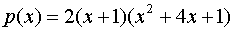.

The roots of the quadratic factor can be found by completing the square or with the quadratic formula.  They turn out to be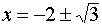.

Now we know that -1 = -tan(45).  We can use the half-angle formula for tangent to see that the remaining roots are -tan(15) and -tan(75). The half-angle formula says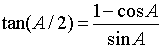.

Taking A = 30 leads to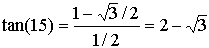,

and with A = 150 we find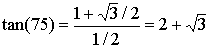.

This shows that the quadratic factor of the polynomial has -tan(15) and -tan(75) as roots.